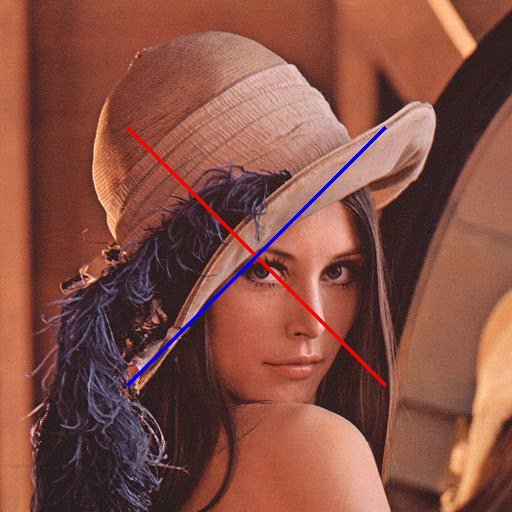• 2021-09-27 10:47:17

imshow(x)
plot([x1,x2],[y1,y2],‘Color’,‘r’,‘LineWidth’,5)
point1 = [x1,y1];
point2 = [x2,y2];matlab
更多相关内容
• NULL 博文链接：https://xurichusheng.iteye.com/blog/2024844
• 代码能够读入的图片中进行直线、折线的绘制，并能够控制端点标记。这个代码弥补了matlab原图中绘制图形的缺陷。matlab plot函数绘制的线段，并没有改变读入图片的数据，直接保存图片的矩阵数据，不会保留绘制的...
• C#图片处理之:在图片上画直线 在图片上画直线比画框更简单。线形的控制还是通过对Pen的设置来实现的。 /**//// /// 在图片上画线 /// /// 原始图 /// 起始点 /// 终止点 /// 线的颜色 /// 线宽 /// 线条样式...

C#图片处理之:在图片上画直线

在图片上画直线比画框更简单。线形的控制还是通过对Pen的设置来实现的。

/** <summary>
/// 在图片上画线
/// </summary>
/// <param name="bmp">原始图</param>
/// <param name="p0">起始点</param>
/// <param name="p1">终止点</param>
/// <param name="RectColor">线的颜色</param>
/// <param name="LineWidth">线宽</param>
/// <param name="ds">线条样式</param>
/// <returns>输出图</returns>
public static Bitmap DrawLineInPicture(Bitmap bmp, Point p0, Point p1, Color LineColor, int LineWidth, DashStyle ds)
...{
if (bmp == null) return null;

if (p0.X == p1.X || p0.Y == p1.Y) return bmp;

Graphics g = Graphics.FromImage(bmp);

Brush brush = new SolidBrush(LineColor);

Pen pen = new Pen(brush, LineWidth);
//pen.Alignment = PenAlignment.Inset;

pen.DashStyle = ds;

g.DrawLine(pen,p0,p1);

g.Dispose();

return bmp;
}

本文来自CSDN博客，转载请标明出处：http://blog.csdn.net/ki1381/archive/2007/08/16/1746255.aspx

展开全文c# nullmatlab
• winform界面上画坐标，360的箭头，曲线等 winform界面上画坐标，360的箭头，曲线等 winform界面上画坐标，360的箭头，曲线等 winform界面上画坐标，360的箭头，曲线等
• Opencv3 的开发环境安装和导入请看 ... 所有的OpenCV3_C++实例代码请看 ...//去掉以上两行代码注释之后就可以不用下面的代码中加上cv::和...//图像上画直线 return 0 ; } 原图像 结果图像

### Opencv3 的开发环境安装和导入请看

http://blog.csdn.net/sileixinhua/article/details/71175748

### 所有的OpenCV3_C++实例代码请看

https://github.com/sileixinhua/OpenCV_C-_tutorials

# 实例

#include "stdafx.h"
#include <opencv2/opencv.hpp>
#pragma comment(lib,"opencv_world330.lib")

//using namespace cv;
//using namespace std;
//去掉以上两行代码注释之后就可以不用在下面的代码中加上cv::和std::
//cv::为OpenCV的函数库
//std::为C++的标准函数库

int Lines() {
const char* filename = "C:\\Code\\FirstOpenCVProgramming\\lena.jpg";

if (mat.empty()) {
throw("Faild open file.");
}

int x0 = mat.cols / 4;
int x1 = mat.cols * 3 / 4;
int y0 = mat.rows / 4;
int y1 = mat.rows * 3 / 4;
//画线的坐标，起始坐标和终止坐标

cv::Point p0 = cv::Point(x0,y0);
cv::Point p1 = cv::Point(x1, y1);
cv::line(mat, p0, p1, cv::Scalar(0, 0, 255), 3, 4);
//画线的函数
p0.y = y1;
p1.y = y0;
cv::line(mat,p0,p1,cv::Scalar(255,0,0),3,4);

cv::imshow("mat",mat);
cv::imwrite("C:\\Code\\FirstOpenCVProgramming\\Lines.jpg", mat);

cv::waitKey();

return 0;
}

int main()
{
//以下的方法如果想运行，直接把前头的注释去掉即可
//运行代码的时候请改掉filename里的地址
//我的opencv是安装在C盘目录下
//一些目录地址请自行修改一下

Lines(); //在图像上画直线

return 0;
}

# 原图像# 结果图像展开全文opencv c语言

您将需要安装

python成像库(PIL). (见

https://pypi.python.org/pypi/PIL).有关安装PIL：

对,安装完毕后,以下代码应该做你所要求的：

import matplotlib.pyplot as plt

import matplotlib.ticker as plticker

try:

from PIL import Image

except ImportError:

import Image

# Open image file

image = Image.open('myImage.tiff')

my_dpi=300.

# Set up figure

fig=plt.figure(figsize=(float(image.size)/my_dpi,float(image.size)/my_dpi),dpi=my_dpi)

# Remove whitespace from around the image

# Set the gridding interval: here we use the major tick interval

myInterval=100.

loc = plticker.MultipleLocator(base=myInterval)

ax.xaxis.set_major_locator(loc)

ax.yaxis.set_major_locator(loc)

ax.grid(which='major', axis='both', linestyle='-')

ax.imshow(image)

# Find number of gridsquares in x and y direction

nx=abs(int(float(ax.get_xlim()-ax.get_xlim())/float(myInterval)))

ny=abs(int(float(ax.get_ylim()-ax.get_ylim())/float(myInterval)))

# Add some labels to the gridsquares

for j in range(ny):

y=myInterval/2+j*myInterval

for i in range(nx):

x=myInterval/2.+float(i)*myInterval

ax.text(x,y,'{:d}'.format(i+j*nx),color='w',ha='center',va='center')

# Save the figure

fig.savefig('myImageGrid.tiff',dpi=my_dpi)

如果在grace_hopper.png示例文件中使用,则会生成以下输出：

展开全文• 线函数，rm是传过来的图片（灰度图像），(a,b),(c,d)为线的端点。 由于默认生成灰度图像全黑，所以这里直接将像素值改为1，白线。 单独处理竖线和横线的情况。 为防止单独沿着一个坐标计算另一个坐标时，...matlab 开发语言
• matlab可以显示一个图片，并用line这些函数显示图片的窗口上画线。但是我一直没找到可以直接原图上画线的方法。 这个matlab程序是根据网上找到的资料改过来的。线速度很快。因为网上找很久也没有找到可以...
• html5 canvas 通过鼠标点击、拖拽、放开出一条直线
• C#的pictureBox绘图，带背景，有橡皮筋效果，实现矩形和直线图片可保存。 开发环境：Visual Studio 2012（.net framework 4.5）
• 主要介绍了python 实现PIL模块在图片画线写字，具有很好的参考价值，希望对大家有所帮助。一起跟随小编过来看看吧
• 回答：Word中绘图缪陈海使用Word作文档时，经常需要用到其绘图功能，这里介绍几种Word中绘图的方法，希望对大家有所帮助。...这一方法虽然能完成大部分的绘图功能，但给图形配文字时却有不...
• 本人做了一个GUI界面，目标是实现axes坐标中导入一张照片，然后可以照片用鼠标圈，然后就可以计算出圈部分占照片总面积的比例；同时也可以用白色覆盖多余部分(可以看成橡皮擦功能吧？)但目前：圈，橡皮...
• ## cv2 在图片上画线

千次阅读 2021-05-11 13:43:46
import cv2 # capture=cv2.VideoCapture(0) img=cv2.imread('../../img/street.jpg',1) cv2.line(img,(0,0),(100,100),color=(255,0,0),thickness=5) # 从（0...# capture.release() # break 注释的代码是视频上画线
• Python+OpenCV 给图像添加直线/矩形/圆/文字1、代码部分2、实现效果 1、代码部分 import cv2 import numpy as np img = np.zeros((640, 640, 3), np.uint8) #创建640*640的零矩阵，3通道 img_green = np.zeros((512...opencv python 计算机视觉
• 转：http://www.eoeandroid.com/thread-114497-1-1.html   画直线效果图：   Main.xml代码 android:orientation="horizontal" andrandroid layout button class import
• C#中画直线，按确定按钮，可以按照预定的路线，画一条直线。
• 用MFC实现 画直线 、曲线 、扇形的功能。并采取多种方式实现，如CDC HDC CClient等等
• 假如现在有如下一张灰度图（图片名为001.jpg），现在要拟合图中的那条白线 代码如下(Matlab2017)： im=imread('001.jpg'); %读取图片 %im=rgb2gray(im); %如果是rgb图片则转为灰度图 [h,w]=size(im); %获取...Matlab 图像处理
• opencv自带的画直线函数： cv2.line(img, pt1, pt2, color[, thickness[, lineType[, shift]]]) img：要画的圆所在的矩形或图像 pt1：直线起点 pt2：直线终点 color：线条颜色，如 (0, 0, 255) 红色，BGR ...python
•opencv 计算机视觉 python
• 截图与线工具，在画线与编辑方面很好用，推荐使用，还是其他功能
• void MainWindow::paintEvent(QPaintEvent *event) { //加载的背景图 QPixmap pixmap(":/new/prefix1/view.jpg");...//画直线 QPainter painter; painter.begin(&pixmap); painter.setPen(Qt::green); painter.qt
• 1在图片上用鼠标进行操作，opencv主要用到setMouseCallback（）函数。 winname 窗口名称 onMouse 鼠标事件的回调函数 userdata 传递给回调函数 还有onMouse函数 event 鼠标事件 x,y 鼠标在图片上的坐标 flags 鼠标...opencv c++...